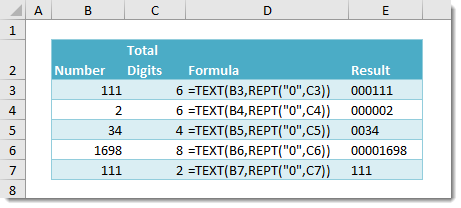## Example

=TEXT(B3,REPT(“0”,C3))

## Generic Formula

=TEXT(Number,REPT(“0”,N))
• Number – This is the number which you want to add leading zeros to.
• N – This is total number of characters you want in the resulting leading zeros number string.

## What It Does

This formula will add leading zeros to a given number up to a specified number of total characters in the result.

## How It Works

REPT(“0”,N) will create a text string with N zeros. In our example, REPT(“0”,6) results in a text string of 6 zeros “000000“.

The TEXT(Number,”000000″) function will then format any given number as a text string with up to 6 characters for leading zeros. In our example, TEXT(111,”000000″) will result in the text string “000111“.